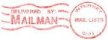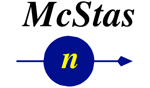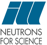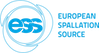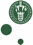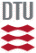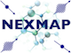McStas: Source_simple Component

# The `Source_simple` Component

A circular neutron source with flat energy spectrum and arbitrary flux

## Identification

• Author: Kim Lefmann
• Origin: Risoe
• Date: October 30, 1997
• Version:(Unknown)
• Modification history:
• KL, October 4, 2001
• Emmanuel Farhi, October 30, 2001. Serious bug corrected.

## Description

```The routine is a circular neutron source, which aims at a square target
centered at the beam (in order to improve MC-acceptance rate).  The angular
divergence is then given by the dimensions of the target.
The neutron energy is uniformly distributed between lambda0-dlambda and
lambda0+dlambda or between E0-dE and E0+dE.
The flux unit is specified in n/cm2/s/st/energy unit (meV or Angs).

This component replaces Source_flat, Source_flat_lambda,
Source_flux and Source_flux_lambda.

Example: Source_simple(radius=0.1, dist=2, focus_xw=.1, focus_yh=.1, E0=14, dE=2)

```

## Input parameters

Parameters in boldface are required; the others are optional.
Name Unit Description Default
radius m Radius of circle in (x,y,0) plane where neutrons are generated. 0.1
yheight m Height of rectangle in (x,y,0) plane where neutrons are generated. 0
xwidth m Width of rectangle in (x,y,0) plane where neutrons are generated. 0
dist m Distance to target along z axis. 0
focus_xw m Width of target .045
focus_yh m Height of target .12
E0 meV Mean energy of neutrons. 0
dE meV Energy half spread of neutrons (flat or gaussian sigma). 0
lambda0 AA Mean wavelength of neutrons. 0
dlambda AA Wavelength half spread of neutrons. 0
flux 1/(s*cm**2*st*energy unit) flux per energy unit, Angs or meV if flux=0, the source emits 1 in 4*PI whole space. 1
gauss 1 Gaussian (1) or Flat (0) energy/wavelength distribution 0
target_index 1 relative index of component to focus at, e.g. next is +1 this is used to compute 'dist' automatically. +1

## Output parameters

Name Unit Description Default
pmul
square
srcArea